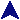Parallel Integration, RegionsSome MPI Examples```#include <stdio.h>
#include <string.h>
#include <stdlib.h>
#include <math.h>
#include <mpi.h>
#include "func.h"

/*
* Find the area to the tolerance.
*/
static double find(double x1, double y1, double x2, double y2, double tol)
{
/* Compute the midpoint from the funcion. */
double midx = (x1 + x2) / 2;
double midy = f(midx);

/* Estimate the midpoint from the y value. */
double midest = (y1 + y2) / 2;

/* See if we're getting close. */
if(fabs(midy - midest) <= tol)
/* Will do.  Compute the area using the midy, since we
found it.  Two trapazoids algebraically simplified. */
return 0.25*(x2-x1)*(y1 + y2 + 2*midy);
else
/* Subdivide and try again. */
return find(x1, y1, midx, midy, tol) +
find(midx, midy, x2, y2, tol);
}

int main(int argc, char **argv)
{
int nreg, myid;
int  namelen;
char processor_name[MPI_MAX_PROCESSOR_NAME];

MPI_Init(&argc,&argv);
MPI_Comm_size(MPI_COMM_WORLD,&nreg);
MPI_Comm_rank(MPI_COMM_WORLD,&myid);

/* Fine argument whine. */
if(argc < 4) {
printf("Usage: %s startx endx tol nreg funcargs*\n",
argv);
MPI_Finalize();
exit(2);
}

#ifdef DEBUG
/* See what we're running on. */
MPI_Get_processor_name(processor_name,&namelen);
printf("Process %d of %d is on %s\n",
myid, nreg, processor_name);
fflush(stdout);
#endif

/* Get the args. */
char *pname = argv;
double start = atof(argv);
double end = atof(argv);
double tol = atof(argv);

argc -= 4; argv += 4;

/* Sanity. */
if(tol < 0.0000000001) {
printf("Tolerance to too small or negative.\n");
MPI_Finalize();
exit(3);
}
if(end < start) {
double t = start;
start = end;
end = t;
}

/* Initialize the function. */
finit(argc, argv);

/* Compute our region based on our rank. */
double inc = (end - start) / nreg;
double startx = start + inc*myid;
double lastx;
if(myid == nreg - 1)
lastx = end;
else
lastx = startx + inc;

#ifdef DEBUG
printf("Proc %d: %g - %g\n", myid, startx, lastx);
#endif

/* Compute for our region. */
double loctot = find(startx, f(startx), lastx, f(lastx), tol);

/* Collect the result. */
double tot;
MPI_Reduce(&loctot, &tot, 1, MPI_DOUBLE, MPI_SUM, 0, MPI_COMM_WORLD);

if(myid == 0)
printf("Integral from %g to %g = %g (tol %g)\n",
start, end, tot, tol);

MPI_Finalize();
return 0;
}

```

This program can be run like this:
 mpiexec -hosts 2 bennet.mc.edu sandbox.mc.edu parallel -120 130 0.000001 5 3 7 1

The number of segments is determined by the number of tasks, which is two. The range is -120 to 130, 0.000001 is the tolerance, and 5 3 7 1 is the polynomial. You can run with more tasks like this:
 mpiexec -hosts 2 bennet.mc.edu 5 sandbox.mc.edu 7 parallel -120 130 0.000001 5 3 7 1